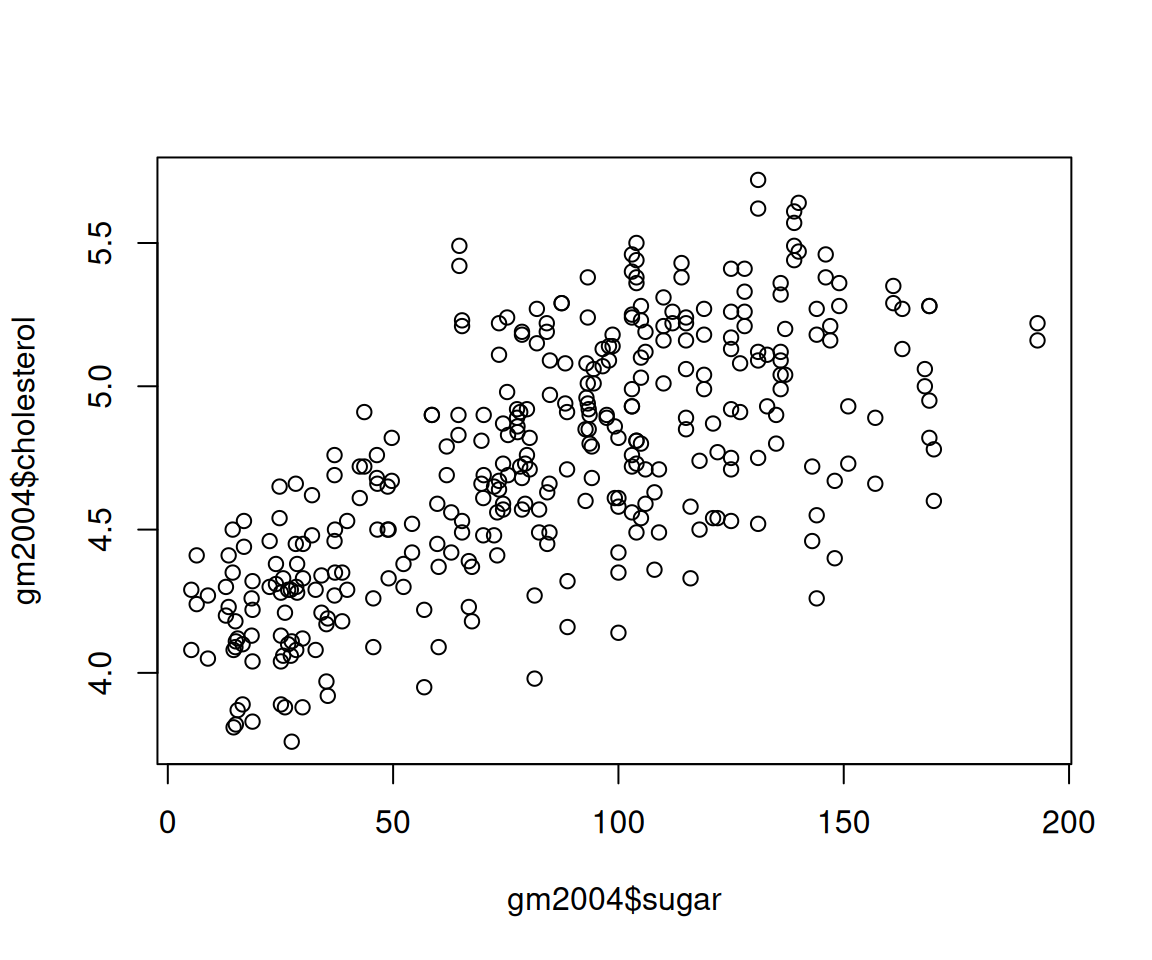## 4.12 Histograms

In Section 4.10, we learned how to use geom_bar() to generate bar charts for visualizing the distributions of discrete variables. You may be wondering, how about visualizing continuous variables? One popular plot is called histograms.

Let’s again use the sahp housing price data set.

### 4.12.1 Using the hist() function

To generate a histogram, you can simply use hist() with the variable as the argument.

library(r02pro)

### 4.12.3 Aesthetics in geom_histogram()

Next, we introduce the aesthetics in histograms, which are very similar to those in bar charts. For example, we can map a variable to the fill aesthetic.

ggplot(data = sahp) +
geom_histogram(mapping = aes(x = sale_price, fill = house_style),
bins = 5)We can see that like the bar chart, the bar for each bin is now divided into sub-bars with different colors. The different colors in each sub-bar correspond to the values of house_style. And the height of each sub-bar represents the count for the cases with the sale_price in this particular bin and the specific value of house_style. Just like geom_bar(), we have a global aesthetic called position, which does position adjustment for different sub-bars. The default position value is again "stack" if you don’t specify it.

a. Stacked bars

ggplot(data = sahp) +
geom_histogram(mapping = aes(x = sale_price, fill = house_style),
position = "stack",
bins = 5)

As expected, we get the same histogram as before.

b. Dodged bars

The second option for position is "dodge", which places the sub-bars beside one another, making it easier to compare individual counts for the combination of a bin of sale_price and house_style.

ggplot(data = sahp) +
geom_histogram(mapping = aes(x = sale_price, fill = house_style),
position = "dodge",
bins = 5)c. Filled bars

Another option for optional is position = "fill". It works like stacking, but makes each set of stacked bars the same height.

ggplot(data = sahp) +
geom_histogram(mapping = aes(x = sale_price, fill = house_style),
position = "fill",
breaks = seq(from = 0, to = 600, by = 100))Just like geom_bar(), the y axis may be labeled as “proportion” rather than “count,” to be more precise. This makes it easier to compare proportions of different values of cut for different bins of price. For example, we can see that the proportion of house_style = "1.5Fin" is highest for the houses with sale price less than 100.

### 4.12.4 Density Estimate Using Histograms

In addition to using histograms to visualize the distribution of a discrete variable, you can also construct a density density of variable using a proper normalization. To generate such a density estimate, you can add y = ..density.. as a mapping in the aes() function in geom_historgram(). Let’s see an example as below.

ggplot(data = sahp) +
geom_histogram(aes(x = sale_price, y = ..density..),
breaks = seq(from = 0, to = 6e2, by = 1e2)) +
scale_y_continuous(breaks = seq(from = 0, to = 7e-3, by = 1e-3))Here, we added the breaks arguments on the bins as well as on the y-axis.

Let’s try to calculate the height of the first bar together. We know that the total area of the bars is 1, agreeing with the definition of density. First, we get the area of the first bar. Then we divide it by the width to get the height.

sale_price_no_na <- na.omit(sahp\$sale_price)
sum(sale_price_no_na < 1e2)/length(sale_price_no_na) #area of the first bar
#>  0.1036585
sum(sale_price_no_na < 1e2)/length(sale_price_no_na) /1e2 #height of the first bar
#>  0.001036585

You can see that the height matches the y axis for the first bar.

### 4.12.5 Exercises

Use the sahp data set to answer the following questions.

1. Create three different histograms on the living area (liv_area) for each of the following settings
• Use 10 bins
• Set the binwidth to be 300
• Se the bins manually to an equally-spaced sequence from 0 to 3500 with increment 500.
1. Create histograms on the living area (liv_area) with 5 bins, and show the information of different kit_qual values in each bar. What conclusions can you draw from this plot?